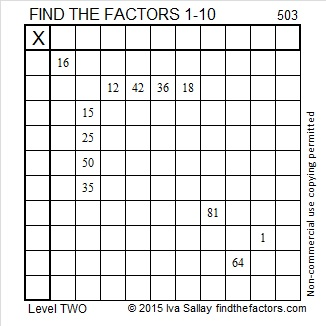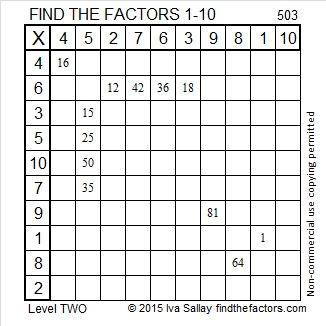# 503 and Level 2

503 = (2^3) + (3^3) + (5^3) + (7^3) which is the sum of the cubes of the first four prime numbers. 503 is the smallest prime number that is the sum of consecutive cubes of prime numbers.

503 is also the sum of three consecutive prime numbers: 163, 167, and 173.Print the puzzles or type the solution on this excel file: 10 Factors 2015-05-25

—————————————————————————————————

• 503 is a prime number.
• Prime factorization: 503 is prime.
• The exponent of prime number 503 is 1. Adding 1 to that exponent we get (1 + 1) = 2. Therefore 503 has exactly 2 factors.
• Factors of 503: 1, 503
• Factor pairs: 503 = 1 x 503
• 503 has no square factors that allow its square root to be simplified. √503 ≈ 22.42766How do we know that 503 is a prime number? If 503 were not a prime number, then it would be divisible by at least one prime number less than or equal to √503 ≈ 22.4. Since 503 cannot be divided evenly by 2, 3, 5, 7, 11, 13, 17, or 19, we know that 503 is a prime number.

—————————————————————————————————This site uses Akismet to reduce spam. Learn how your comment data is processed.